Next: 2.2 Tetrahedral Grids Up: 2. Grid Types Previous: 2. Grid Types

Subsections

# 2.1 Ortho Grids

The simplest way for meshing a region is to cut the simulation area into layers of different thickness. After cutting in all three directions of space, we get an ortho grid. For the grid representation, only the elements that lie within the simulation domain are relevant (Figure 2.1).

Figure 2.1: Two-dimensional ortho grids along axes-parallel and non-parallel geometry edges.Axes-parallel geometry edges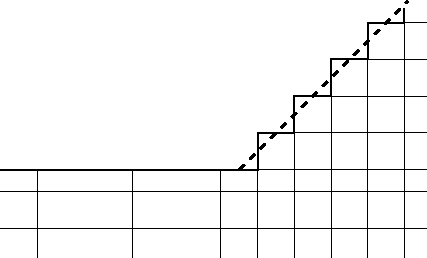Non-parallel geometry edges, with required refinement along them

If all edges of the geometry of the structure are parallel to the coordinate axes, we will find an acceptable mesh for this domain. However, with complex geometrical structures the limits of this method are quickly reached. Basically, inserting a new point in an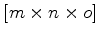points wide grid, where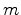,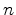, and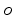denote the number of ticks in the three coordinate directions, will produce a grid with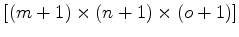points. This may pose an unacceptable computational burden in terms of CPU-time and memory consumption.

Especially with non-planar geometries, there is no way to reproduce such non-planar surfaces. They must be approximated by steps in the geometry, as sketched in Figure 2.1 for a two dimensional example of an ortho grid. To achieve the desired resolution of those edges which are non-parallel to the coordinate axes these lines must be approximated by small steps. A negative side effect of this method is that the amount of grid points will rise dramatically. Each additional coordinate tick will result in an unacceptable number of unwanted grid points.

## 2.1.1 Mixed Grids

As a solution to this dilemma, it can be useful to allow triangular or tetrahedral elements, too. This is shown in Figure 2.2. Grid lines, which are needed in one region only, can terminate inside the simulation domain. At the termination points, rectangles (cuboids) with additional points on the sides arise. As such rectangles (cuboids) usually are not supported, they must be split into triangles (tetrahedrons) to satisfy the connectivity. However, if triangles or tetrahedrons are provided, they can also be provided at the geometry edges, which is shown in the region near the fat boundary edges of Figure 2.2. This generally will reduce the amount of grid points while better approximating the geometry.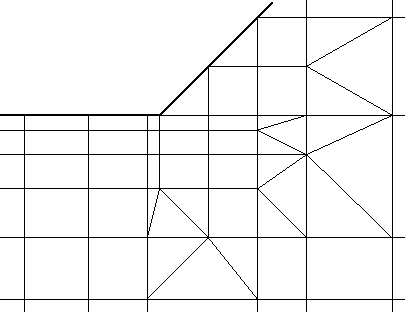Furthermore, since ortho grids can be split into triangles (or tetrahedra for three dimensions), there is no need to store rectangles any longer. Each rectangle can be split in two triangles, each cuboid can be split into five or six tetrahedrons. As a side effect, this decomposition fulfills the Delaunay criterion, which is relevant for certain numerical schemes as mentioned previously. Adding special terminating lines criteria within these generated triangles, a valid triangular (tetrahedral) Delaunay mesh can be built.

## 2.1.2 Providing Attributes

Ortho grids are a common way to provide attributes, such as dopant concentrations. On each point of theortho grid several attribute values can be stored. The actual simulation is performed on a separate simulation grid, which can be handled independently from these attribute grids. This has the big advantage that the layout of the attribute and simulation grid can be customized to their individual requirements. Regridding, coarsening, and refining the simulation grid will not alter the attributes and no information loss of the primary attribute data is caused by a change of the simulation grid. Otherwise, the resolution of the attributes can be tuned arbitrarily, without an increase of the simulation times. Before starting the simulation, the attribute values of the ortho grid are interpolated to the simulation grid. In general, attributes are often provided on different grids than the simulation grid.Next: 2.2 Tetrahedral Grids Up: 2. Grid Types Previous: 2. Grid Types

J. Cervenka: Three-Dimensional Mesh Generation for Device and Process Simulation